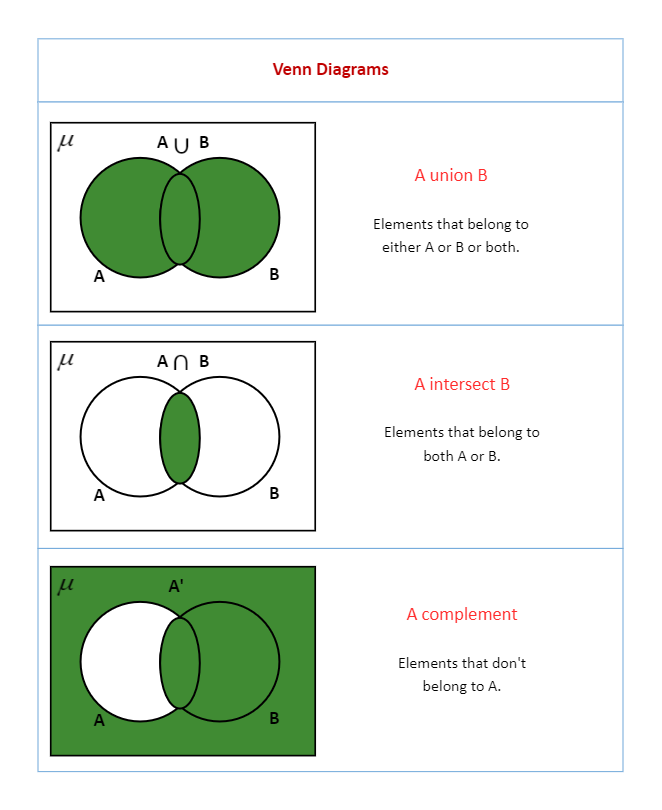Template Community / Venn Diagram Math

# Venn Diagram MathCommunity Helper
Published on 2022-08-08
Edit OnlineThis Venn Diagram Math is a diagram that depicts the elements of a set or group. It is a diagram representing all of the logical relationships between a finite set of sets or groups. It is also recognized as a set diagram or a logic diagram. Venn Diagram Math has been used in introductory logic curriculums and elementary-level educational plans worldwide since the mid-twentieth century. The overlapping areas can also demonstrate where two otherwise dissimilar contexts share similarities. A Venn diagram can be organized in various ways, but the most common are overlapping circles. Each circle represents a set, which could be ideas, concepts, numbers, or objects.
Tag
venn diagram
Venn Diagram Collection
education Venn Diagram
Report
1
95PostRecommended Templates